#### Neyman Allocation

When you specify the ALLOC=NEYMAN option in the STRATA statement, PROC SURVEYSELECT allocates the total sample size among the strata in proportion to stratum sizes and stratum variances. Neyman allocation is a special case of optimal allocation (described in the section Optimal Allocation), where the costs per unit are the same for all strata. For Neyman allocation, the proportion of the total sample size for stratum h is computed as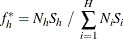If you specify the total sample size n in the SAMPSIZE= option in the PROC SURVEYSELECT statement, the procedure computes the target sample size for stratum h as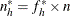. The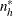are converted to integer sample sizes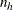by using a rounding algorithm that requires the sum of the stratum sizes to equal n. The final sample sizesare required to be at least 1, or at least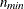if you specify a minimum sample size in the ALLOCMIN= option in the STRATA statement. For without-replacement selection methods, the final sample sizes must not exceed the stratum sizes.